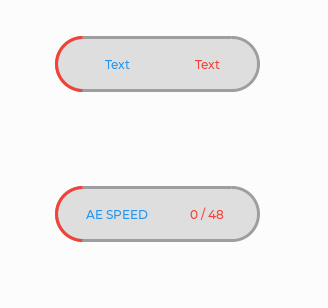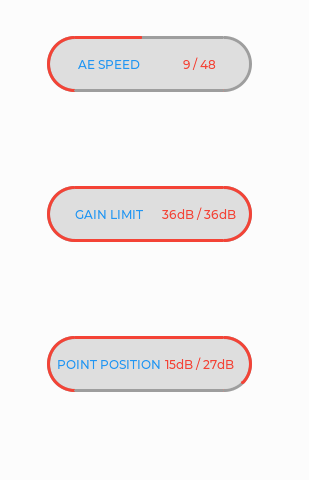# Can multiple parameters be carried in the event callback function

Hi,
As a novice, I have two questions to ask:

1.Can multiple parameters be carried in the event callback function?

For example, in the figure,label,btn can they be used as parameters in the function?

2.Can the gauge be changed to the following figure?lvgl version: 8.3.0

Thank’s.

First of all in next time use [code] (```) block to post source code.
For answer to your first question need more information `event_handler_q5_1()` assigned to which object?
Second. As I know, the gauge can be only round or arc.

First question: I want to add the event callback function to a button, but there is no place to add parameters when adding it.Can I add some parameters, such as static void event_handler_q1(lv_event_t * e,lv_obj_t *label_2,lv_obj_t *slider1)

``````static void event_handler_q1(lv_event_t * e)
{
lv_event_code_t code = lv_event_get_code(e);
if(code == LV_EVENT_CLICKED) {
i_1=++i_1;
LV_LOG_USER("i_1: %d\n", i_1);
char buf;
lv_snprintf(buf,sizeof(buf),"%s / %s",*(arr+i_1),"1/30");
lv_label_set_text(label_2,buf);
if(0<=(i_1*(100/10))&&(i_1*(100/10))<40)
{
lv_slider_set_value(slider1,(i_1*(100/10)),0);

}else if(40<=(i_1*(100/10))&&(i_1*(100/10))<60)
{
lv_slider_set_value(slider1,40,0);
lv_arc_set_value(arc2, (i_1*(100/10)));

}else if(60<=(i_1*(100/10))&&(i_1*(100/10))<=100)
{
lv_slider_set_value(slider1,40,0);
lv_arc_set_value(arc2, 60);
lv_slider_set_value(slider2,(60+(100-(i_1*(100/10)))),0);
}
}
}

``````

The second question: I want to know whether there is an official widgets that can achieve this effect by changing its style, except for the combination of widgets?

If you planning to work with only CLICK event, then assign handler to only CLICK event:

``````lv_obj_add_event_cb(btn_1, event_handler_q1, LV_EVENT_CLICKED, NULL);
``````

Next. `label_2` is inside this button? Then you can get handle of this label by request childs of button:

``````lv_obj_t *btn = lv_event_get_target(e);
lv_obj_t *lbl = lv_obj_get_child(btn, 0);

lv_label_set_text(lbl, .... );
``````

Slider you can pass as param to event:

``````lv_obj_add_event_cb(btn_1, event_handler_q1, LV_EVENT_CLICKED, slider1);
``````

end then get this param from event:

``````static void event_handler_q1(lv_event_t * e)
{
lv_obj_t *slider = lv_event_get_user_data(e);
...
}
``````

or you can allocate static array of ojects and pass it as param to event:

``````static lv_obj_t ctrls = { label_2, slider_1 };

static void event_handler_q1(lv_event_t * e)
{
lv_obj_t *ctrls = lv_event_get_user_data(e);
lv_obj_t *label  = ctrls;
lv_obj_t *slider  = ctrls;
...
}
``````

What? What kind of effect do you want?

This widgets is composed of two sliders, two labels, two arcs, and a button. I wonder if there is a simpler way to implement it?I also have a question, can this param pass different types of parameters at the same time?

``````static void event_handler_q1(lv_event_t * e)
{
lv_event_code_t code = lv_event_get_code(e);
if(code == LV_EVENT_CLICKED) {
i_1=++i_1;
LV_LOG_USER("i_1: %d\n", i_1);
char buf;
lv_snprintf(buf,sizeof(buf),"%s / %s",*(arr+i_1),"1/30");
lv_label_set_text(label_2,buf);
if(0<=(i_1*(100/10))&&(i_1*(100/10))<40)
{
lv_slider_set_value(slider1,(i_1*(100/10)),0);

}else if(40<=(i_1*(100/10))&&(i_1*(100/10))<60)
{
lv_slider_set_value(slider1,40,0);
lv_arc_set_value(arc2, (i_1*(100/10)));

}else if(60<=(i_1*(100/10))&&(i_1*(100/10))<=100)
{
lv_slider_set_value(slider1,40,0);
lv_arc_set_value(arc2, 60);
lv_slider_set_value(slider2,(60+(100-(i_1*(100/10)))),0);
}
}
}
``````

such as int i_1 and lv_obj_t *slider1 and char arr?

I don’t understand what must be in result. Can you draw different states?

you can pass pointer to any data. C Pointers